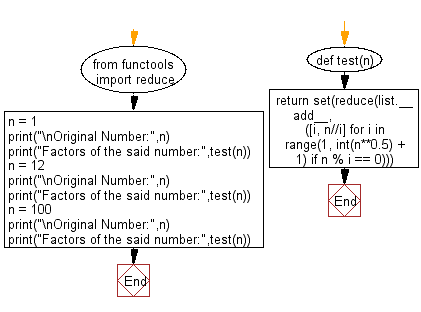﻿ Python: Find all the factors of a given natural number - w3resource# Python: Find all the factors of a given natural number

## Python Basic - 1: Exercise-132 with Solution

Write a Python program to find all the factors of a given natural number.

Factors:
The factors of a number are the numbers that divide into it exactly. The number 12 has six factors:
1, 2, 3, 4, 6 and 12 If 12 is divided by any of the six factors then the answer will be a whole number. For example:
12 ÷ 3 = 4

Sample Solution

Python Code:

``````#Source https://bit.ly/3w492zp

from functools import reduce

def test(n):
([i, n//i] for i in range(1, int(n**0.5) + 1) if n % i == 0)))

'''
sqrt(x) * sqrt(x) = x. So if the two factors are the same, they're both
the square root. If you make one factor bigger, you have to make the other
factor smaller. This means that one of the two will always be less than or
equal to sqrt(x), so you only have to search up to that point to find one
of the two matching factors. You can then use x / fac1 to get fac2.

The reduce(list.__add__, ...) is taking the little lists of [fac1, fac2]
and joining them together in one long list.

The [i, n/i] for i in range(1, int(sqrt(n)) + 1) if n % i == 0 returns
a pair of factors if the remainder when you divide n by the smaller one
is zero (it doesn't need to check the larger one too; it just gets that
by dividing n by the smaller one.)

The set(...) on the outside is getting rid of duplicates, which only
happens for perfect squares. For n = 4, this will return 2 twice, so
set gets rid of one of them.
'''
n = 1
print("\nOriginal Number:",n)
print("Factors of the said number:",test(n))
n = 12
print("\nOriginal Number:",n)
print("Factors of the said number:",test(n))
n = 100
print("\nOriginal Number:",n)
print("Factors of the said number:",test(n))
``````

Sample Output:

```Original Number: 1
Factors of the said number: {1}

Original Number: 12
Factors of the said number: {1, 2, 3, 4, 6, 12}

Original Number: 100
Factors of the said number: {1, 2, 4, 100, 5, 10, 50, 20, 25}
```

Flowchart:## Visualize Python code execution:

The following tool visualize what the computer is doing step-by-step as it executes the said program:

Python Code Editor:

Have another way to solve this solution? Contribute your code (and comments) through Disqus.

What is the difficulty level of this exercise?

Test your Programming skills with w3resource's quiz.

﻿

## Python: Tips of the Day

What is the difference between Python's list methods append and extend?

append: Appends object at the end.

```x = [1, 2, 3]
x.append([4, 5])
print (x)
```

Output:

```[1, 2, 3, [4, 5]]
```

extend: Extends list by appending elements from the iterable.

```x = [1, 2, 3]
x.extend([4, 5])
print (x)
```

Output:

```[1, 2, 3, 4, 5]
```

Ref: https://bit.ly/2AZ6ZFq